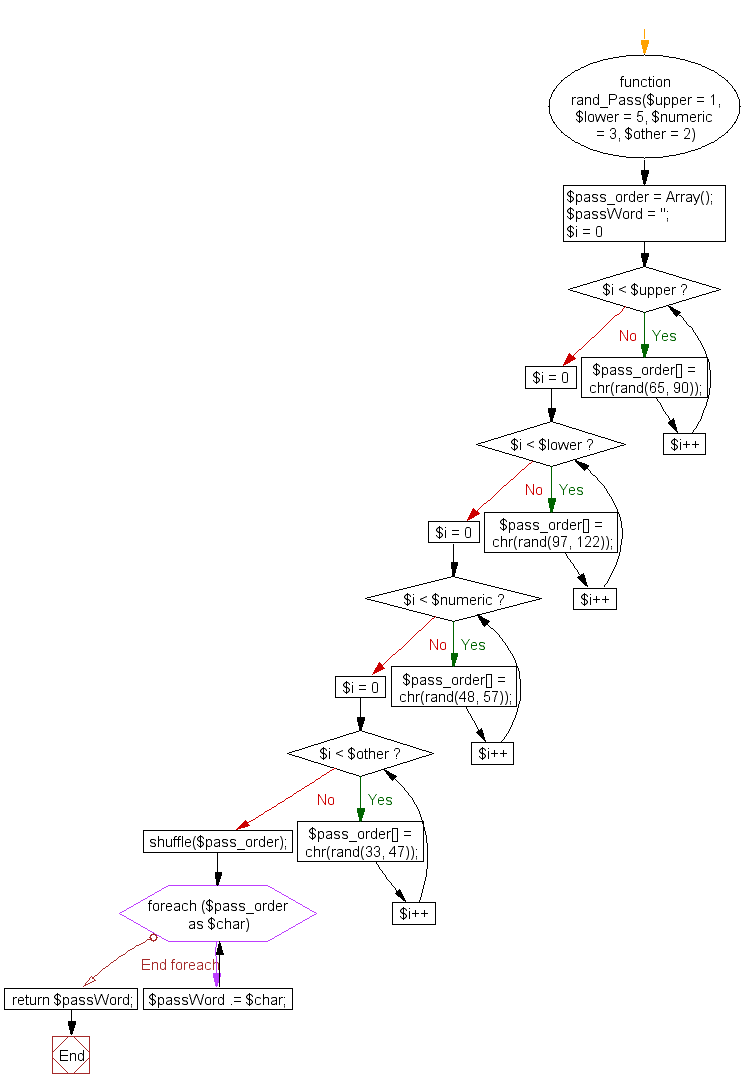﻿ PHP Array Exercise: Generate a random password using shuffle function - w3resource# PHP Array Exercises : Generate a random password using shuffle() function

## PHP Array: Exercise-27 with Solution

Write a PHP function to generate a random password (contains uppercase, lowercase, numeric and other) using shuffle() function.

Sample Solution:

PHP Code:

``````
<?php
function rand_Pass(\$upper = 1, \$lower = 5, \$numeric = 3, \$other = 2) {

\$pass_order = Array();

for (\$i = 0; \$i < \$upper; \$i++) {
\$pass_order[] = chr(rand(65, 90));
}
for (\$i = 0; \$i < \$lower; \$i++) {
\$pass_order[] = chr(rand(97, 122));
}
for (\$i = 0; \$i < \$numeric; \$i++) {
\$pass_order[] = chr(rand(48, 57));
}
for (\$i = 0; \$i < \$other; \$i++) {
\$pass_order[] = chr(rand(33, 47));
}

//using shuffle() to shuffle the order
shuffle(\$pass_order);

foreach (\$pass_order as \$char) {
}
}
?>
```
```

Sample Output:

```Generated Password : h1'1#h7Gqfy
```

Flowchart:PHP Code Editor:

What is the difficulty level of this exercise?

﻿

## PHP: Tips of the Day

PHP: Extract numbers from a string

```\$str = 'In My Cart : 11 12 items';
preg_match_all('!\d+!', \$str, \$matches);
print_r(\$matches);
```